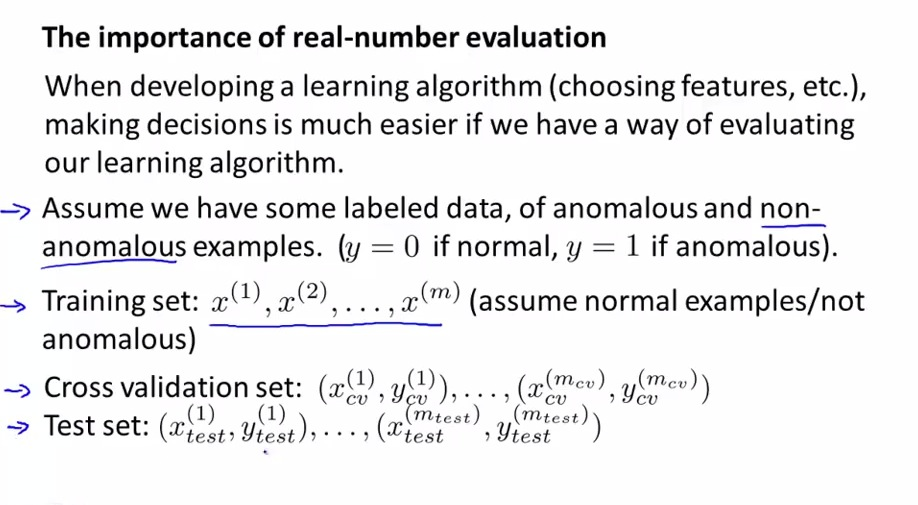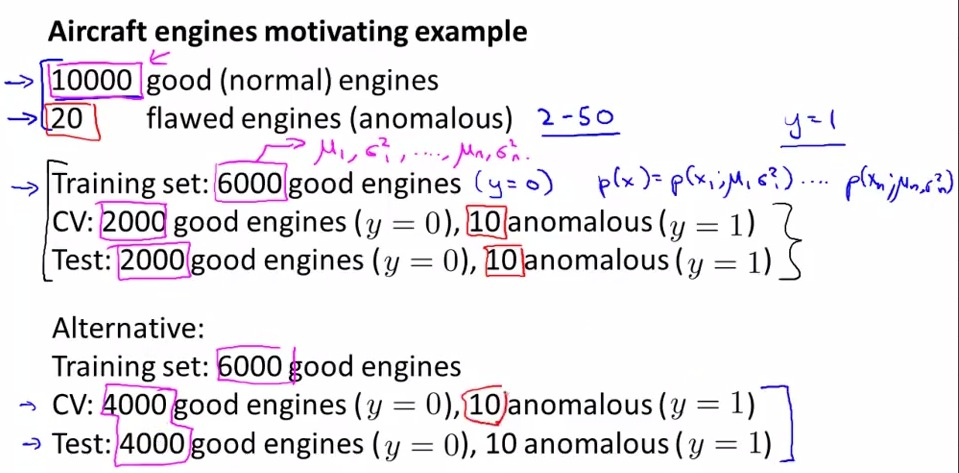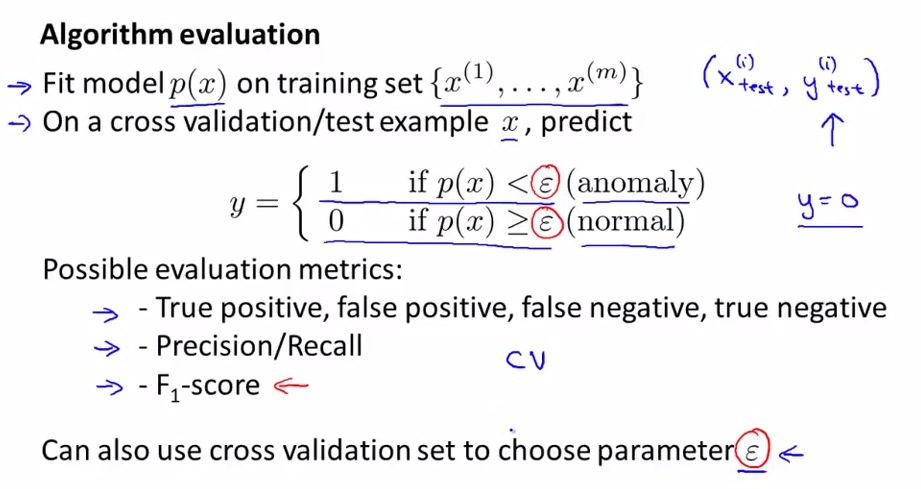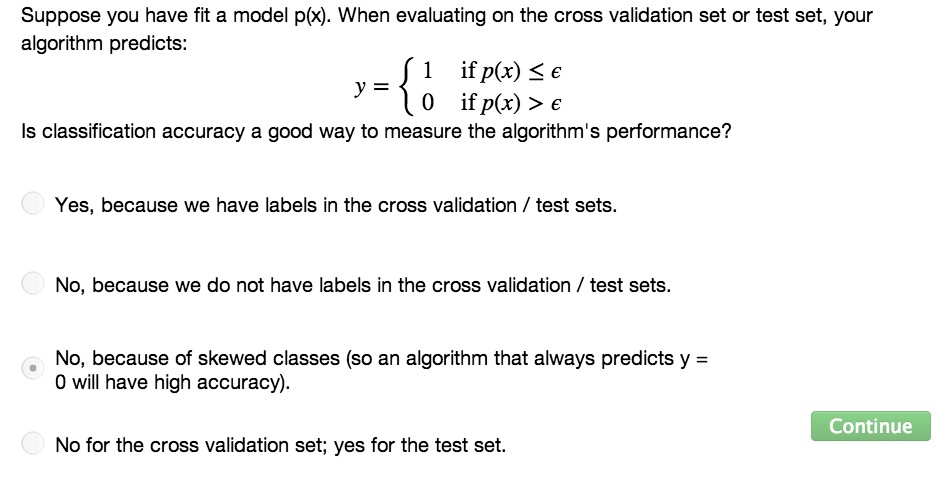• In this video we're going to implement specific application for Anomaly Detection
• This lesson also help us for evaluating Anomaly Detection• When building learning algorithm for our specific purposes, often decision will be made easier if we have a way of evaluating it
• So we may want to labeled our data as anomolous(y = 1) or normal (y= 0) just to test whether our features is good or bad features. or the feature is the deciding point the example is anomalous, or the feature is not worth to implement.
• Then at first we can treat all examples are normal ( let few anomalous examples slip by)
• Then we separate cv and test set that only have anomalous examples.• the number of anomalous typical is 2-50
• For examples above, suppose we have 10000 normal and 20 anomaly. Let it slip, and dividing the examples into 60% training set, 20% cv, 20% test set 10 of each cv and test are put as anomalous.
• In future, we may eventually know some ML practitioners that use same examples for training set, CV, and Test set as shown in the alternative case above. IT'S LESS RECOMMENDED, and not a good ML practice.• given training set, in cv, test, predict whether examples anomaly or not
• similar as supervised learning give labeled data
• Mind that because most of the data are normal examples, the graph itself tend to shape skew-class (y = 0) for classification
• consider y = 0 all the time will give too high accuracy, so classification may not recommended
• The good way for evaluating it, is choose one of the alternative in possible evaluation metrics. We may want to choose epsilon manually, and test it in cv test that maximize F-1 score, or at least it does well in cv test
• When epsilon doing well in cv test, and learning behave correctly, finally test the algorithm in the test set• Process and evaluate Anomaly Detection in number F-1 score will be make time more efficient
• We also learned how to use labeled data for Anomaly Detection, similar as supervised learning
• Next, when to choose Anomaly Detection wisely, or when choosing Anomaly Detection vs Supervised Learning